## Decimals Numbers Story Sums

#### 1. Kelly bought 0.5 litre of milk and 0.25 litre of juice. Gloria bought 0.65 litre of milk and 0.8 litres of juice. a) How many litres of beverages did the two girls buy altogether? b) How many more litres of beverages did Gloria buy than Kelly?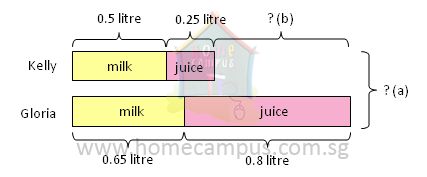a)   0.5  +  0.25  +  0.65  +  0.8  =  2.2
The two girls bought 2.2 litres of beverages altogether.

b)   0.5  +  0.25  =  0.75
Kelly bought 0.75 litre of beverages.

0.65  +  0.8  =  1.45
Gloria bought 1.45 litres of beverages.

1.45  −  0.75  =  0.7
Gloria bought 0.7 litre more beverages than Kelly.

#### 2. In a certain competition, the participants had to swim a distance of 800 m, run a distance of 5.6 km and cycle a distance of 12.3 km. What was the total distance a participant had to cover? Give your answer in km.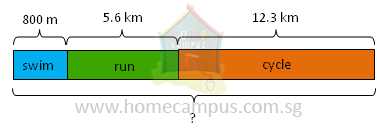0.8  +  5.6  +  12.3  =  18.7
The total distance a participant had to cover was 18.7 km.
1000 m  =  1 km
800 m  =  0.8 km

#### 3. Tom is 1.61 m tall. Jerry is 45 cm shorter than Tom. How tall is Jerry?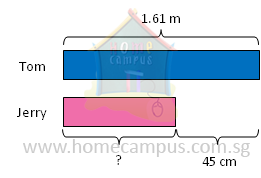1.61  −  0.45  =  1.16
Jerry is 1.16 m tall.
100 cm  =  1 m
45 cm  =  0.45 m

#### 4. Ryan and Bryan went to a strawberry farm. Ryan picked 1.25 kg of strawberries. Bryan picked twice as much strawberries as Ryan. a) Find the mass of the strawberries that Bryan picked. b) Find the mass of the strawberries that the two boys picked altogether.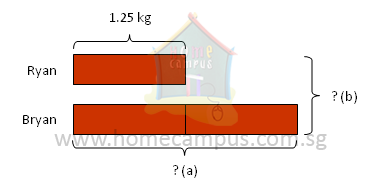a)   1 unit   ->  1.25 kg
2 units  ->  1.25 kg  ×  2
=  2.5 kg
Bryan picked 2.5 kg of strawberries.

b)   3 units  ->  1.25 kg  ×  3
=  3.75 kg
The two boys picked 3.75 kg of strawberries altogether.

#### 5. The area of a rectangle is 21 cm2. If the length of the rectangle is 6 cm, what is its breadth?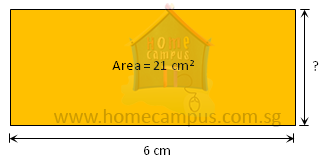21  ÷  6  =  3.5
The breadth of the rectangle is 3.5 cm.

#### 6. The mass of 6 identical paper weights is 2.16 kg. Find the mass of 8 such paper weights. Round off your answer to the nearest kilogram.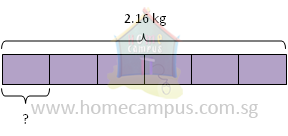2.16  ÷  6  =  0.36
The mass of 1 paper weight is 0.36 kg.

0.36  ×  8  =  2.88
2.88 kg   ≈  3 kg  (when rounded off to the nearest kilogram)
The mass of 8 paper weights is about 3 kg.

#### 7. Angie paid \$22.45 for a bottle of orange juice and 4 packets of cake mix. The bottle of orange juice cost \$2.25. How much did each packet of cake mix cost?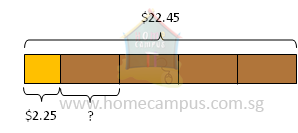\$22.45  −  \$2.25  =  \$20.20

\$20.20  ÷  4  =  \$5.05
Each packet of cake mix cost \$5.05.

#### 8. Shop A, Shop B and Shop C sell roses. Shop A sells one rose for \$2.65. Shop B sells it for 20 ¢ more than Shop A and 35 ¢ less than Shop C. If Kate bought 7 roses from Shop C, how much did she spend?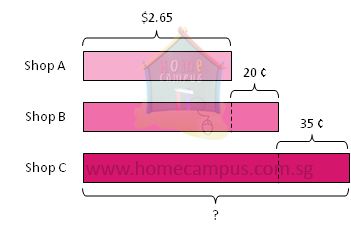\$2.65  −  \$0.20  =  \$2.85
Shop B sells 1 rose for \$2.85.
100 ¢  =  \$1
20 ¢  =  \$0.20
\$2.85  −  \$0.35  =  \$3.20
Shop C sells 1 rose for \$3.20.

\$3.20  ×  7  =  \$22.40
Kate spent \$22.40.
35 ¢  =  \$0.35

#### 9. A fruiterer had 300 apples. After keeping 15 apples for himself, he packed the remaining apples in bags of 5. If he sold each bag at \$3.25, how much money did he collect?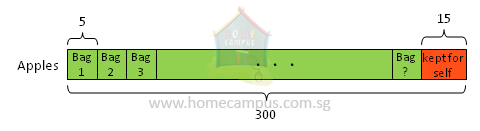300  −  15  =  285
He packed 285 apples.

285  ÷  5  =  57